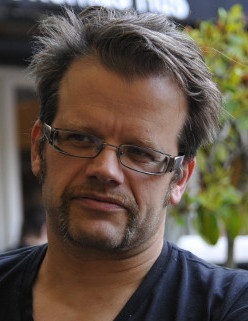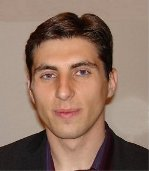# ESQC lectures

### Except for autodidactic purposes, you are not allowed to use these notes without written consent from the authors.Daniel Crawford (Virginia Tech)
Coupled Cluster Theory (3 lectures) [ pdf 1/4   pdf 2/4   pdf 3/4   pdf 4/4 ]
Fundamentals of single-reference coupled cluster theory, including both second-quantized and diagrammatic expositions, size extensivity, perturbative corrections, excited states, analytic gradients, and strategies for efficient computer implementations.Trygve Helgaker (Oslo)
Molecular properties (4 lectures) [ pdf 1/4   pdf 2/4   pdf 3/4   pdf 4/4 ]
The analytical calculation of molecular properties with emphasis on first- and second-order properties. Variational Lagrangian for non-variational electronic-structure models. The 2n+1 and 2n+2 rules. Molecular gradients and molecular Hessians. Molecular structure and vibrational frequencies. The electronic Hamiltonian in an electromagnetic field. Gauge dependence and London orbitals. NMR shielding and indirect nuclear spin-spin coupling constants. Geometry optimizations. Newton and quasi-Newton methods. Minima and saddle points.Wim Klopper (Karlsruhe)
Basis Sets, Integrals and SCF Methods (4 lectures) [pdf 1/4   pdf 2/4   pdf 3/4   pdf 4/4 ]
Basic tools and techniques of rigorous molecular electronic structure theory, fundamental for the treatment of molecular properties. The electronic Schrödinger equation. Slater determinants and the Hartree-Fock or self-consistent field (SCF) approximation. The concepts of closed and open shell states, molecular orbitals (MOs) and spin orbitals, restricted and unrestricted SCF procedures, Koopmans' and Brillouin's theorem. Introduction of a basis set (LCAO) expansion for the MOs and the Roothaan-Hall equations. Techniques for the evaluation of integrals over Gaussian functions and direct SCF procedures. Discussions of recent developments.Fred Manby (Bristol)
QM/MM and other hybrid models in chemistry (2 lectures) [pdf 1/2   pdf 2/2 ]
Introduction to the QM/MM embedding method. Issues of efficiency, and effects beyond electrostatics. Polarizable embedding. Polarizable continuum methods. Combinations of electronic structure methods through embedding methods based on density-functional theory.Frank Neese (Mülheim)
Algorithm design (1 lecture) [ pdf 1/1 ]
Efficient implementation of quantum chemical equations. Do's and don'ts of quantum chemical programming. Obtaining exact numbers in the most efficient way vs. obtaining approximate numbers efficiently
Approximation methods (1 lecture)
The lecture will cover a range of approximation methods that are in widespread use in quantum chemistry and will discuss their advantages and disadvantages. Special attention will be given to numerical thresholding and controlled precision. Applications to the self-consistent field (Hartree-Fock & DFT) as well as MP2 will be briefly touched upon.
Local correlation (1 lecture) [pdf 1/1]
Various approaches to calculating the dynamic correlation energy for large molecules: incremental methods, domain based local schemes, pair natural orbital approaches
General aspects of computational chemistry (1 lecture)
Design issues encountered in planning an actual computational chemistry study. Incentive for thinking about the goals of the computational study, but not a set of 'carved in stone' recipes. Actual exampleJeppe Olsen (Aarhus)
The Multiconfigurational Approach (3 lectures) [pdf 1/1]
Near degeneracies in molecular systems: transition states in chemical reactions, excited states, molecules with competing valence structures. The MCSCF wave function and energy expression. The multiconfigurational SCF equations. The Newton-Raphson and super-CI methods. Complete and restricted active spaces. Different types of MCSCF wave functions. Excited states and transition properties. Multiconfigurational second order perturbation theory. Multireference Configuration Interaction techniques.
Introduction to Response Theory (1 lecture) [pdf 1/1]
This lecture gives a short introduction to response functions and their use to describe the properties of ground and excited states. The response functions are introduced as terms in the expansion of the the time-development of an expectation value for a Hamiltonian including a time-dependent perturbation. It is shown that the linear response function provides information about excitation energies and transition moments. The quasi-energy is introduced and the equality between the stationarity of this energy and the time-dependent Schrödinger equation is discussed, which allows the use of the quasi-energy to obtain the time-development for approximate wave functions and densities. A brief overview of the various approximate response models is given, including their computational complexity and limitations.Trond Saue (Toulouse)
Second Quantization (2 lectures) [ pdf 1/2   pdf 2/2 ]
The formalism of second quantization provides an alternative representation of quantum mechanics, that is useful for orbital based models. In second quantization Slater determinants are represented by occupation number vectors in an abstract vector space, the Fock space. Operators are represented by linear combinations of products of creation and annihilation operators. The use of finite basis sets leads to deviations from the usual commutators between operators.
Relativistic Quantum Chemistry (3 lectures) [ pdf 1/1   ]
Basics of relativistic effects in the electronic structure of atoms and molecules. Relativistic theory of many-electron systems. Dirac equation and Dirac-Coulomb-Breit equation. Transformations of the Dirac equation to two-component form. Effective Core Potentials. Spin-orbit coupling in molecules. Applications of relativistic methods in heavy-element chemistry.Julien Toulouse (Paris)
Density Functional Theory (3 lectures) [pdf 1/1]
1. Basic DFT: Hohenberg-Kohn theorem, Levy’s constrained-search formulation, Kohn-Sham method, Practical calculations in an atomic basis, spin DFT.
2. More advanced topics in DFT: Exchange and correlation holes, Adiabatic connection, Scaling relations, Fractional electron numbers and frontier orbital energies, Derivative discontinuity, Fundamental gap, Excitation energies.
3. Usual approximations for the exchange-correlation energy: Local-density approximation, Generalized-gradient approximations, Meta-generalized-gradient approximations, Hybrid approximations, Double-hybrid approximations, Range-separated hybrid approximations, Semiempirical dispersion corrections.Per-Olof Widmark (Lund)
Mathematical Tools in Quantum Chemistry (2 lectures) [pdf 1/1]
This course gives an introduction/refresher in basic nomenclature and definitions of spaces and operators of importance in quantum chemistry, and their properties. Convergence/divergence of series and of iterative processes is analyzed. Modern methods for eigenvalue problems are described, in particular for CI applications where dimensions can be very large. Similarly, solution methods for large linear and non-linear equation systems are presented.Peter Taylor (Tianjin)
Symmetry and Quantum-chemical Calculations (1 lecture) [ pdf 1/1 ]
In this lecture we review various aspects of molecular symmetry relevant to quantum-chemical calculations. After considering the various symmetry properties of the Hamiltonian, we focus first on the use of point-group symmetry and projection operators to obtain symmetry-adapted functions. We then briefly visit spin and the connection between second quantization and the general linear group, before concluding with some discussion of electron configurations that ties together spin, spatial, and permutational symmetries.